# Homework H5.O - Fa23

 Problem statement Solution video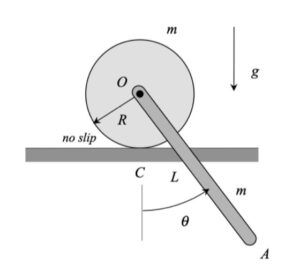Discussion and hints: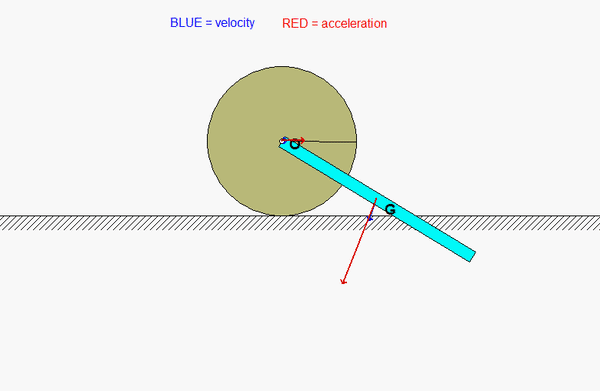Recall the following four-step plan outline in the lecture book and discussed in lecture:

Step 1: FBDs
Draw individual free body diagrams for the bar and the disk.

Step 2: Kinetics (Newton/Euler)
Write down the Newton/Euler equations for the disk and for the bar based on your FBDs above.

Step 3: Kinematics
You need to use the rigid body acceleration equations to relate the accelerations of O and the center of mass of the bar, G, through the angular acceleration of the bar. Also, you need to relate the acceleration of O to the angular acceleration of the disk.

Step 4: Solve
Solve your equations for the angular accelerations of the bar and the disk.

Any questions?

# Homework H5.P - Fa23

 Problem statement Solution videoAny questions??

DISCUSSIONWith the bar welded to the disk, the two bodies move as a single rigid body. That is, they have the same angular velocity as the disk rolls without slipping. For position 2 shown below, the velocities of O (center of mass of the disk) and G (center of mass of the bar) are in opposite directions and appear to have nearly equal speeds. Do they have exactly the same speed? (Consider the location of the instant center for the disk as it rolls.)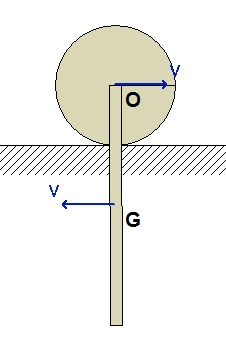As always, we should follow the four-step plan for solving this problem.

STEP 1: FBD. We will be using the work/energy equation to solve this problem since we are looking for a change in speed for a change in position. Based on earlier recommendations, we will make the choice of our system BIG, including the disk and bar together.
STEP 2: Kinetics (here, work/energy). The total kinetic energy of the system shown in your FBD above is that of the bar + disk. For the disk, it is recommended that you choose the no-slip point as your reference point for the KE. For the bar, you could use either C or G for the reference point for its KE.  Be reminded that your choice of reference points dictates the mass moments of inertia that are to be used for the rigid bodies. Be sure to identify the datum line for the gravitational potential energy, and use this in writing down this potential. Is energy conserved?
STEP 3: Kinematics. As described above, the IC for the bar+disk is the no-slip point C.
STEP 4: Solve

# Homework H5.M - Fa23

 Problem statement Solution video

Note: Please plug in the given values and leave your final answer in terms of omega only.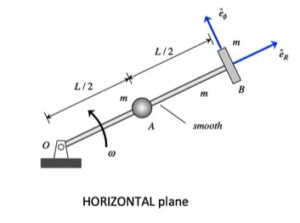DISCUSSION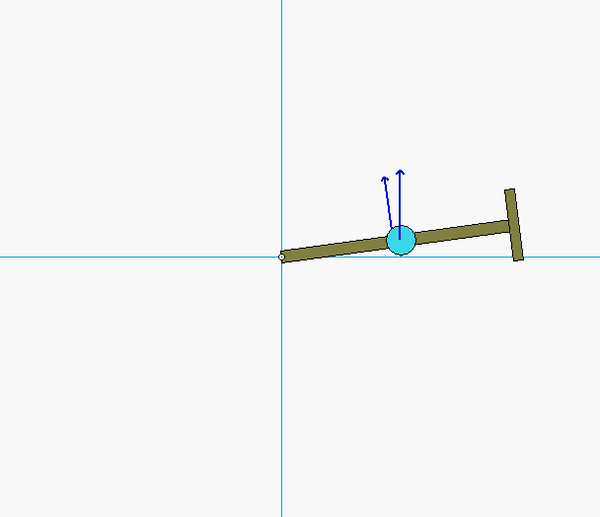Four-step plan

Step 1: FBD
Draw a free-body diagram particles A and B, along with the bar, altogether. Is angular momentum for this system conserved? Why, or why not?

Step 2: Kinetics - Angular impulse/momentum
Write down the angular momentum for A, B and the bar individually, and then add together. For a particle A, for example, recall that angular momentum is given by HO = m rA/O x vA.  For a rigid body, HO = IOω. You will also need the coefficient of restitution equation relating the radial (normal components here) components of velocity of A and B during impact.

Step 3: Kinematics
For writing down the velocity of points A and B, a polar description is recommended. For example, vA = R_dot eR + Rωeθ.

Step 4: Solve
Solve your equations from Steps 2 and 3 for the velocity of P. Do not forget that A will have both radial and transverse components of velocity.

# Homework H5.N - Fa23

 Problem statement Solution video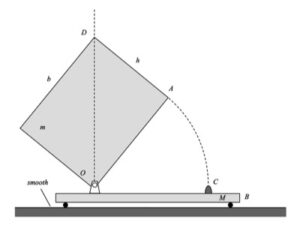Discussion and hintsThe four-step plan:

1. FBD: It is recommended that you draw a free body diagram of the plate and cart combined.
2. Kinetics: Your FBD should show that the summation of forces in the direction of motion for the cart (call that the x-direction) is zero; therefore, linear momentum in the x-direction is conserved for ALL time. Also note that energy is conserved up to the point of impact. During impact, energy is not conserved; however, you are given the coefficient of restitution (COR) for the impact.
3. Kinematics: Write down the rigid body velocity equation relating the motion of points O and the plate's center of mass G. Here you will use the fact that the cart moves only in the x-direction.
4. Solve

# Homework H5.K - Fa23

 Problem statement Solution videoDiscussion

.The animation shown above demonstrates the relationship between the translation velocity of the disk's center A and the angular velocity of the disk. Recall that the disk is initially projected to the right with an initial velocity of A and a counterclockwise rotation rate; that is, the disk is given a "backspin" on release.

As the disk slides, the friction with the floor does two things: it slows down the translational motion of the disk, and exerts a clockwise moment on the disk reducing the rotational rate of the disk. At some point the rotation rate changes from counterclockwise (positive) to clockwise (negative). And shortly after that, slipping ceases to exist between the disk and the floor. Rolling without slipping starts at the point where the plots of velocity of A and angular velocity of the disk go "flat".

HINT: As always, we should follow the four-step plan for solving this problem.
STEP 1: FBD. Here, draw a free body diagram of the disk.
STEP 2: Kinetics (here, linear and angular impulse momentum equations).
STEP 3: Kinematics. Slipping ceases when the velocity of the contact point on the disk with the ground goes to zero. At that point: vA = ω x rA/C, where C is the point on the disk in contact with the ground.
STEP 4: Solve

# Homework H5.L - Fa23

 Problem statement Solution videoDiscussion and hints: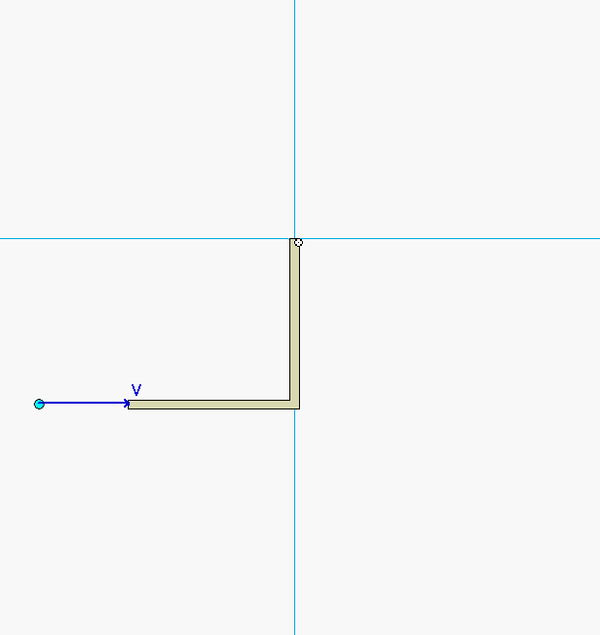Note that the animation above is for a generic set of parameter values that may or may not be the ones assigned for the problem this semester.

Recall the following four-step plan outline in the lecture book and discussed in lecture:

Step 1: FBDs
Draw a single free body diagram for the system made up of bar OA and particle P, combined.

Step 2: Kinetics (impulse/momentum)

• From you FBD above, what can you say about the moments acting about the fixed point O in your system? What consequence does this have on the angular momentum about point O for the system?
• Use the coefficient of restitution, COR, equation relating the "n-components" of velocity for P and end A of the bar. (You may want to review Section 4.C of the lecture book in regard to impacts and the COR.)

Step 3: Kinematics
Note that bar OA rotates about point O. What does that say about the direction of the velocity of point A on the bar after impact? Study the animation above, and the freeze-frame images below of the motion. Do your kinematics agree with what you see about the direction of the velocity of A?Step 4: Solve
Solve your equations above for the angular speed of bar OA.

Any questions?

# Homework H5.I - Fa23

 Problem statement Solution video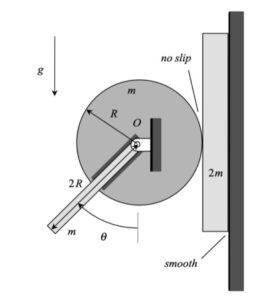Discussion and hints:Recall the following four-step plan outline in the lecture book and discussed in lecture:

Step 1: FBDs
Draw a single free body diagram for the system made up of the disk, bar and block, combined.

Step 2: Kinetics (work/energy)

• Write down the kinetic expressions for the three bodies in the system, individually, and then add those together to find the total KE for the system of your FBD.
• Do the same for the potential energies: write down the PEs for each body individually and add together.
• Also, based on your FBD above, which, if any force or couple, does nonconservative work on the system in your FBD? Determine work for such a force/couple.

Step 3: Kinematics
Since the bar is welded to the disk, the angular velocities of the bar and disk are the same. The no-slip condition at the point of contact of the disk and block enforces a constraint between the rotation rate of the disk and the translational speed of the block. What is that constraint?

Step 4: Solve
Solve your equations above for the angular velocity of the disk.

Any questions?

# Homework H5.J - Fa23

 Problem statement Solution videoAny questions??

DISCUSSIONFour-step plan

Step 1: FBD
Draw a free-body diagram for the plate.

Step 2: Kinetics - Work/energy
Write down the kinetic energy and potential energy expressions for the plate, along with the work done on the disk by non-conservative forces. The instant center, IC, is fairly easy to locate for Position 2 - you are able to write down the KE for the plate as Tplate = 0.5*IICω2, if you choose to do so. The work done by F is equal to U = FsD, where sD is the distance traveled by corner D of the plate.

Step 3: Kinematics
The easiest approach here is to use the location of the IC for the plate.Step 4: Solve
Solve your equations from Steps 2 and 3 for the angular speed of the plate.

# Homework H5.G - Fa23

 Problem statement Solution videoAny questions??

Four-step plan

Step 1: FBD
Draw a free-body diagram for the disk.

Step 2: Kinetics - Work/energy
Write down the kinetic energy and potential energy expressions for the disk, along with the work done on the disk by non-conservative forces. Note that point C is the instant center (zero velocity point) for the disk. Because of this, you are able to write down the KE for the disk as Tdisk = 0.5*ICω2, if you choose to do so. The work done by F is equal to U = FsA, where sA is the distance traveled by end A of the cable.

Step 3: Kinematics
Here you need to relate the distance traveled by point O to the distance traveled by end A of the cable (these are not the same). In doing this, recall that C is the instant center of the disk as it rolls without slipping.

Step 4: Solve
Solve your equations from Steps 2 and 3 for the speed of point O.

# Homework H5.H - Fa23

 Problem statement Solution videoDiscussion and hints:Recall the following four-step plan outline in the lecture book and discussed in lecture:

Step 1: FBDs
Draw a single free body diagram for the system made up of block A, the disk and the pulley combined.

Step 2: Kinetics (work/energy)

• Write down the kinetic expressions for the block, disk and pulley individually, and then add those together to find the total KE for the system of your FBD. For each KE expression, recall that your reference point for the rotational component needs to be either the center of mass of the body, or a fixed point on the rigid body. You might consider using the no-slip, rolling contact point C as your reference point for the disk.
• Do the same for the potential energies: write down the PEs for each body individually and add together.
• Also, based on your FBD above, which, if any, force does nonconservative work on the system in your FBD? Determine the work done by such a force.

Step 3: Kinematics
Note that the instant center (IC) for the disk is the no-slip contact point C. (Carefully study either the animation above - you can actually see the IC from this!) Locating this IC is critical for you in setting up and using the kinematics for this problem. What is the speed of point B on the disk as compared to the disk's center E? (Refer back to C being the IC for the disk.) See the freeze-frame image below, and compare the speed of B with that of A.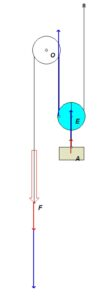Step 4: Solve
Solve your equations above for the speed of block A.

Any questions?